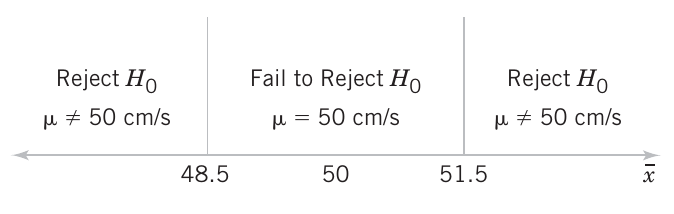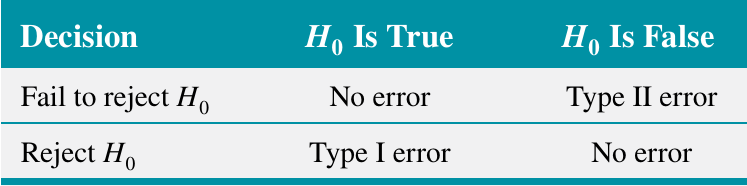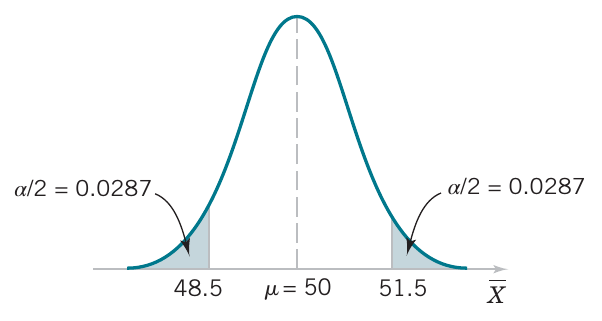# Hypothesis testing

04/14/2021

## Instructions:

Use the left and right arrow keys to navigate the presentation forward and backward respectively. You can also use the arrows at the bottom right of the screen to navigate with a mouse.

FAIR USE ACT DISCLAIMER:
This site is for educational purposes only. This website may contain copyrighted material, the use of which has not been specifically authorized by the copyright holders. The material is made available on this website as a way to advance teaching, and copyright-protected materials are used to the extent necessary to make this class function in a distance learning environment. The Fair Use Copyright Disclaimer is under section 107 of the Copyright Act of 1976, allowance is made for “fair use” for purposes such as criticism, comment, news reporting, teaching, scholarship, education and research.

## Outline

• The following topics will be covered in this lecture:
• A review of hypothesis testing
• Decision criteria for hypothesis testing
• Duality with confidence intervals
• Types of errors
• Power of statistical tests
• P values

## Hypothesis testing – a review

• Formally, we will define
Statistical Hypothesis
A statistical hypothesis is a statement about the parameters of one or more populations.
• Because we use probability distributions to model populations, a statistical hypothesis may also be thought of as a statement about the probability distribution of a random variable.

• The hypothesis will usually involve one or more parameters of this distribution.

• For example, consider the air crew escape system described last time.

• Suppose that we are interested in the burning rate of the solid propellant.

• Burning rate is a random variable that can be described by a probability distribution.

• Suppose that our interest focuses on the mean burning rate (a parameter of this distribution).

• Specifically, we are interested in deciding whether or not the mean burning rate is $$50$$ centimeters per second.

• We may express this formally as

\begin{align} H_0∶& \mu = 50 \text{ centimeters per second}\\ H_1∶& \mu \neq 50 \text{ centimeters per second} \end{align}

• $$H_0$$ is known as the null hypothesis and $$H_1$$ is known as the alternative hypothesis.

### Hypothesis testing – a review

• In hypothesis testing, the null and alternative hypotheses have special meanings philosophically and in the mathematics.

• We cannot generally “prove” a hypothesis to be true;

• generically, we will assume that the true population parameter is unobservable.
• Instead, we can only determine if a hypothesis seems unlikely enough to reject;

• this is similar to finding that our proposed parameter value was in far-fewer confidence intervals than predicted by the procedure.
• To begin such a test formally, we need to first make some assumption about the true parameter.

• This always takes the form of assuming the null hypothesis $$H_0$$.
• The null hypothesis $$H_0$$ will always take the form of an equality, or an inclusive inequality.

• That is, we take

\begin{align} H_0: & \theta \text{ is } (= / \leq / \geq) \text{ some proposed value}. \end{align}

• In our example, we wrote

\begin{align} H_0∶ & \mu = 50 \text{ centimeters per second}. \end{align}

### Hypothesis testing – a review

• The contradictory / competing hypothesis is the alternative hypothesis, written

\begin{align} H_1: & \theta \text{ is } (\neq / > / <) \text{ some proposed value} \end{align}

• In our example, we wrote

\begin{align} H_1∶ & \mu \neq 50 \text{ centimeters per second}. \end{align}

• Once we have formed a null and alternative hypothesis:

\begin{align} H_0: & \theta \text{ is } (= / \leq / \geq) \text{ some proposed value}\\ H_1: & \theta \text{ is } (\neq / > / <) \text{ some proposed value} \end{align}

• we use the sample data to consider how likely or unlikely it was to observe such data with the proposed parameter.

• If the sample doesn't seem to fit the proposed parameter value, we deem the null hypothesis unlikely.
• If the null hypothesis is sufficiently unlikely, we reject the null hypothesis in favor of the alternative hypothesis.

• However, if the evidence (the sample) doesn't contradict the null hypothesis, we tentatively keep this assumption.

• This has not proven this assumption, it has only said that the hypothesis is not unlikely given our evidence.
• In our example, we would say either:

1. we reject the null hypothesis of $$H_0∶ \mu = 50$$ in favor of the alternative $$H_1: \mu \neq 50$$; or
2. we fail to reject the null hypothesis of $$H_0:\mu = 50$$.

### Hypothesis testing – a review

• In our example, the alternative hypothesis specifies values of $$\mu$$ that could be either greater or less than 50 centimeters per second;

• therefore, it is called a two-sided alternative hypothesis.
• In some situations, we may wish to formulate a one-sided alternative hypothesis, as in

\begin{align} H_0∶ & \mu \geq 50\text{ centimeters per second} \\ H_1∶ & \mu < 50\text{ centimeters per second} \end{align}

• or

\begin{align} H_0∶ & \mu \leq 50\text{ centimeters per second} \\ H_1∶ & \mu > 50\text{ centimeters per second} \end{align}

• The above situations have an exact analogy with one-sided confidence bounds, similar to the two-sided test and the two-sided confidence interval.

• We will now elaborate on the meaning of determining if a hypothesis is sufficiently unlikely.

• This is directly related to the value $$\alpha$$ we used as a rate of failure for confidence intervals.

## Decision citeria for hypothesis testing

• In our working example, we are considering

\begin{align} H_0∶& \mu = 50 \text{ centimeters per second}\\ H_1∶& \mu \neq 50 \text{ centimeters per second} \end{align}

• Suppose that the standard deviation of the burning rate is a known $$\sigma = 2.5$$ centimeters per second and that the burning rate has a normal distribution.

• Assuming the null hypothesis, and with a sample of $$n = 10$$ specimens,

$\overline{X} \sim N\left(\mu, \frac{\sigma^2}{n}\right) = N\left(50,0.625\right)$

• A value of the sample mean $$\overline{x}$$ that falls close to the hypothesized value of $$\mu = 50$$ centimeters per second (relative to the spread) does not conflict with the null hypothesis that the true mean $$\mu$$ is really $$50$$ centimeters per second.

• On the other hand, a sample mean that is considerably different from $$50$$ centimeters per second is evidence in support of rejecting the null hypothesis.

• The sample mean in this test is what is called a test statistic.

• The test statistic is what we will use to evaluate how likely or unlikely the observed data is based on our assumed null hypothesis.
• The sample mean is of course a random variable and can take on many different values.

### Decision citeria for hypothesis testing

• However, assuming the null hypothesis, and with a sample of $$n = 10$$ specimens,

$\overline{X} \sim N\left(50, 0.625\right)$

• we can compute the probability of observing various values of the test statistic, i.e.,

$P(\overline{X}\leq \overline{x}_l) \text{ or } P(\overline{X} \geq \overline{x}_u).$ where $$\overline{x}_l$$ and $$\overline{x}_u$$ may be some lower and upper bound.

• For sake of example, let's suppose that observing a sample mean that is $$1.9$$ standard deviations away from the center of the sampling distribution would be highly surprising:

\begin{align} & &-1.9 &= \frac{\overline{x}_l - 50}{.79} & & & 1.9 &= \frac{\overline{x}_u - 50}{.79}\\ \Leftrightarrow & & \overline{x}_l& \approx 48.5 & & & \overline{x}_u &\approx 51.5 \end{align}

• We can compute the associated probability

se = 2.5 / sqrt(10)
mu = 50
1 - pnorm(51.5, mean=mu, sd=se) + pnorm(48.5, mean=mu, sd=se)

 0.05777957


### Decision citeria for hypothesis testing

• In the last slide, we proposed the criterion that observing a sample mean $$1.9$$ standard deviations away from the hypothesized center $$\mu=50$$ to be reason to question the hypothesis.
• This corresponded to a decision that if $$48.5 \leq \overline{x} \leq 51.5,$$ we will not reject the null hypothesis $$H_0$$, and if either $\overline{x} < 48.5 \text{ or }\overline{x} > 51.5,$ we will reject the null hypothesis in favor of the alternative hypothesis $$H_1$$.
• This is illustrated to the right.Courtesy of Montgomery & Runger, Applied Statistics and Probability for Engineers, 7th edition

• The values of $$\overline{x}$$ that are less than $$48.5$$ and greater than $$51.5$$ constitute the critical region for the test;
• all values that are in the interval $48.5 \leq \overline{x} \leq 51.5$ form a region for which we will fail to reject the null hypothesis.
• By convention, this is usually called the acceptance region.
• The boundaries between the critical regions and the acceptance region are called the critical values – in our example, the critical values are $$48.5$$ and $$51.5$$.
• It is customary to state conclusions relative to the null hypothesis $$H_0$$.
• Therefore, we reject $$H_0$$ in favor of $$H_1$$ if the test statistic falls in the critical region and fails to reject $$H_0$$ otherwise.

### Decision citeria for hypothesis testing – types of errors

• Our decision process is based on the random outcome of the test statistic, so that even if an outcome seems unlikely, we may come to a false conclusion based on observing a low-probability event.
• There are two possible wrong conclusions we can make in this decision process:
1. we may reject the null hypothesis when this is actually true;
2. we may fail to reject the null hypothesis when this is actually false.
• In our example, the true mean burning rate of the propellant could be equal to $$50$$ centimeters per second;
• yet for the specimens that are tested, we could observe a value of the test statistic $$\overline{x}$$ that falls into the critical region.Courtesy of Montgomery & Runger, Applied Statistics and Probability for Engineers, 7th edition

• We would then reject the null hypothesis $$H_0$$ in favor of the alternate $$H_1$$ when, in fact, $$H_0$$ is really true.
• This type of wrong conclusion is called a type I error.
• Type I Error
Rejecting the null hypothesis $$H_0$$ when it is true is defined as a type I error.

### Decision citeria for hypothesis testing – types of errors

• Now suppose that the true mean burning rate is different from 50 centimeters per second
• yet for the specimens that are tested, we could observe a value of the test statistic $$\overline{x}$$ that falls into the acceptance region.Courtesy of Montgomery & Runger, Applied Statistics and Probability for Engineers, 7th edition

• We would then fail to reject the null hypothesis $$H_0$$ when, in fact, $$H_0$$ is really false.
• This type of wrong conclusion is called a type II error.
Type II Error
Failing to reject the null hypothesis $$H_0$$ when it is false is defined as a type II error.
• A schematic of this hypothesis testing decision process is given in the right:Courtesy of Montgomery & Runger, Applied Statistics and Probability for Engineers, 7th edition

• Based on these two possible errors, we can define different probabilistic criteria that will attempt to handle these risks of incorrect decisions.
• It turns out that the rate of failure of confidence intervals is the same as the probability of type I error.
• Probability of Type I Error
$\alpha = P(\text{type I error}) = P(\text{reject }H_0\text{ when }H_0\text{ is true})$

### Decision citeria for hypothesis testing – types of errors

• If we consider the last statement,

Probability of Type I Error
$\alpha = P(\text{type I error}) = P(\text{reject }H_0\text{ when }H_0\text{ is true}).$ This is also called the significance level of the hypothesis test.
• the dual relationship with confidence intervals can be understood as follows.

• Suppose we produce a $$(1-\alpha)\times100\%$$ confidence interval for the unknown true mean $$\mu$$ of a studied population,

$\left( \overline{x} - \sigma_\overline{X} z_\frac{\alpha}{2} , \overline{x} + \sigma_\overline{X} z_\frac{\alpha}{2}\right)$ based on some sample.

• Suppose that we have a working hypothesis that $$\tilde{\mu}$$ is the true population mean, i.e.,

\begin{align} H_0 &: \mu = \tilde{\mu} \\ H_1 &: \mu \neq \tilde{\mu} \end{align}

• We can considering using the confidence interval as our decision criterion for the hypothesis test:

1. if $$\tilde{\mu}\in \left( \overline{x} - \sigma_\overline{X} z_\frac{\alpha}{2} , \overline{x} + \sigma_\overline{X} z_\frac{\alpha}{2}\right)$$ then we fail to reject the null hypothesis, as $$\tilde{\mu}$$ is a plausible value with $$(1-\alpha)\times 100\%$$ confidence.
2. if $$\tilde{\mu}\notin \left( \overline{x} - \sigma_\overline{X} z_\frac{\alpha}{2} , \overline{x} + \sigma_\overline{X} z_\frac{\alpha}{2}\right)$$ then we reject the null hypothesis, as $$\tilde{\mu}$$ is not a plausible value with $$(1-\alpha)\times 100\%$$ confidence.

### Decision citeria for hypothesis testing – types of errors

• Let's recall the definition of $$\alpha$$:

Probability of Type I Error
$\alpha = P(\text{type I error}) = P(\text{reject }H_0\text{ when }H_0\text{ is true})$
• Suppose that the null hypothesis is true, i.e, $$\tilde{\mu} = \mu$$, yet we find that

$\tilde{\mu} \notin \left( \overline{x} - \sigma_\overline{X} z_\frac{\alpha}{2} , \overline{x} + \sigma_\overline{X} z_\frac{\alpha}{2}\right).$

• If $$H_0$$ is actually true, then concluding that $$\tilde{\mu}$$ is not a reasonable value for $$\mu$$ is precisely a type I error.

• If we have constructed a $$(1-\alpha)\times 100\%$$ confidence interval, the rate at which

$\tilde{\mu} \notin \left( \overline{X} - \sigma_\overline{X} z_\frac{\alpha}{2} , \overline{X} + \sigma_\overline{X} z_\frac{\alpha}{2}\right)$ with respect to infinite replications is precisely the rate of failure, $$\alpha$$.

• Therefore, we have the equivalence:

$(1-\alpha)\times 100\% \text{ confidence} \Leftrightarrow \alpha = P(\text{type I error}).$

• The above relationship expresses the duality of confidence intervals and hypothesis tests.

• This explains, in part, why t.test() computes both a confidence interval and hypothesis test simultaneously;

• the two procedures are formally equivalent.

### Decision citeria for hypothesis testing – types of errors

• If we consider how we constructed the confidence interval, the duality makes sense once again.
• In our earlier example, we had $$H_0: \mu = 50$$ and $$H_1 : \mu \neq 50$$.
• We define our decision criterion in that we will reject the null hypothesis if $$\overline{x}$$ lies outside of a stated interior region around the assumed $$\mu=50$$.Courtesy of Montgomery & Runger, Applied Statistics and Probability for Engineers, 7th edition

• This region is constructed by considering the distribution for the test statistic, i.e., let’s suppose for simplicity that $$\sigma$$ is known and $\overline{X} \sim N\left(\mu, \sigma^2\right).$
• Assuming $$\mu =50$$, we can compute critical values $$z_\frac{\alpha}{2}$$ for which \begin{align} P\left(\overline{X} < \overline{x}_l\right) = P\left(\overline{X} < 50 - \sigma_\overline{X} z_\frac{\alpha}{2}\right) & = \frac{\alpha}{2}\\ P\left(\overline{X} > \overline{x}_u\right) = P\left(\overline{X} > 50 - \sigma_\overline{X} z_\frac{\alpha}{2}\right) & = \frac{\alpha}{2}. \end{align}
• Recall that $$\sigma_\overline{X} z_\frac{\alpha}{2}$$ is precisely the radius of the confidence interval.
• Therefore, the confidence interval centered at $$\overline{x}$$ does not contain $$\mu=50$$ if and only if \begin{align} \overline{x} < 50 - \sigma_\overline{X} z_\frac{\alpha}{2} && \text{ or } \overline{x} > 50 + \sigma_\overline{X} z_\frac{\alpha}{2} \end{align}
• I.e., we reject the null hypothesis if and only if the test statistic is observed beyond the critical values for the sampling distribution.

### Decision citeria for hypothesis testing – types of errors

• In our running example, we had $$z_\frac{\alpha}{2} = 1.9$$ standard deviations, i.e.,
alpha <- 2 * (1 - pnorm(1.9))
alpha

 0.05743312

• where \begin{align} P\left(\overline{X} < \overline{x}_l\right) = P\left(\overline{X} < 48.5\right) \approx P\left(\overline{X} < 50 - \sigma_\overline{X} 1.9\right) & = \frac{\alpha}{2}\\ P\left(\overline{X} > \overline{x}_u\right) = P\left(\overline{X} > 41.5 \right) \approx P\left(\overline{X} > 50 - \sigma_\overline{X} 1.9\right) &= \frac{\alpha}{2}. \end{align}

• Up to some small approximation errors (done in the book)

se = 2.5 / sqrt(10)
mu = 50
1 - pnorm(51.5, mean=mu, sd=se) + pnorm(48.5, mean=mu, sd=se)

 0.05777957

• we see how we found the probability of a type I error in multiple ways.

### Decision citeria for hypothesis testing – types of errors

• In evaluating a hypothesis-testing procedure, it is also important to examine the probability of a type II error, which we denote by $$\beta$$.
Probability of Type II Error
$\beta = P(\text{type II error}) = P(\text{failing to reject }H_0\text{ when }H_0\text{ is false}).$ The complementary probability, $$1- \beta$$ is called the power of the hypothesis test.
• To calculate $$\beta$$, we must have a specific alternative hypothesis;

• that is, we must have a particular value of $$\mu$$.
• This is because, the unknown, true alternative hypothesis for $$\mu$$ will determine the sampling distribution for $$\overline{X}$$.

• For example, suppose that it is important to reject the null hypothesis

$H_0 : \mu = 50$

whenever the mean burning rate $$\mu$$ is greater than $$52$$ centimeters per second or less than $$48$$ centimeters per second.

• Assuming that the true sampling distribution is centered at $$\mu=52$$ or $$\mu=48$$, we can determine the probability of a type II error $$\beta$$;

• we will assume that the decision rule uses the false hypothesis $$\mu=50$$, without knowing the true parameter.
• We will estimate how the test procedure will work probabilistically if we wish to reject $$H_0$$, for a true mean value of $$\mu = 52$$ or $$\mu = 48$$.

### Decision citeria for hypothesis testing – types of errors

• Because of symmetry, it is necessary to evaluate only one of the two cases;
• we will find the probability of failing to reject the null hypothesis $$H_0: \mu = 50$$ centimeters per second when the true mean is $$\mu = 52$$ centimeters per second.